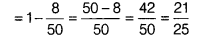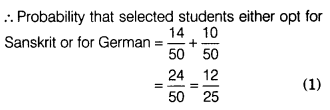# In class IX of 50 students second language opted by the students is as follows

In class IX of 50 students second language opted by the students is as follows Sanskrit -14, Japanese -08, French -12, Urdu -6.
Rest of them opted for German.
A student is selected at random. Find the probability that the student
(i) opts for French.
(ii) does not opts for Japaness.
(iii) Either opts for Sanskrit or German.

∵ Total numbers of students = 50 [given]
∴ Total numbers of trial = 50
(i) Probability that a student selected is opts French language = 12/50 = 6/25
(ii) Probability that a student selected does not opt for Japaness
= 1 - selected students opts Japaness(iii) Probability that selected student either opts for Sanskrit or for German = Probability of students opts Sanskrit + Probability of students opts German
∴ Number of students who opted German
= 50-(14+ 8+12 + 6)
= 50-40 = 10